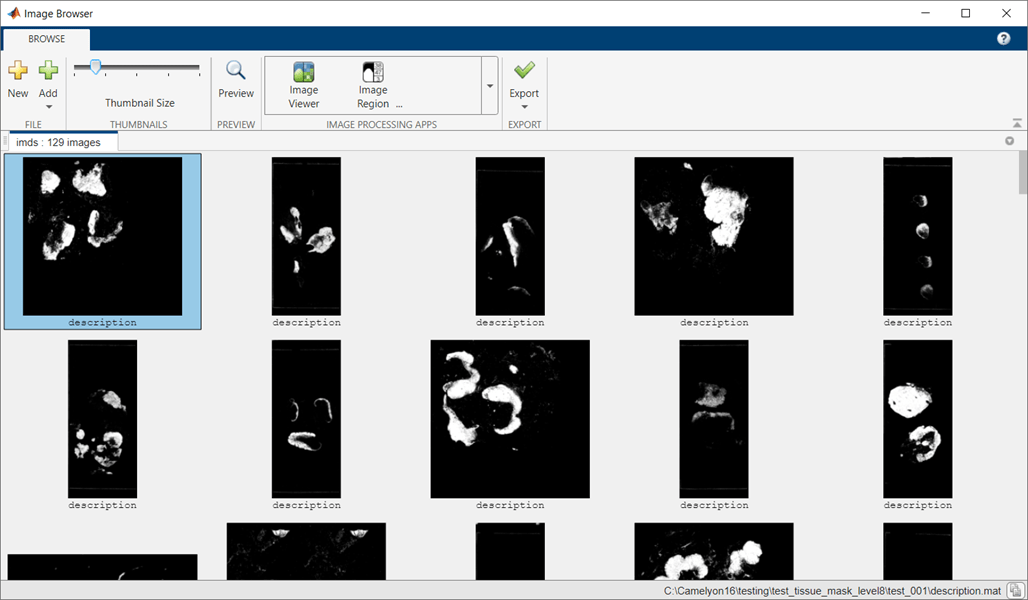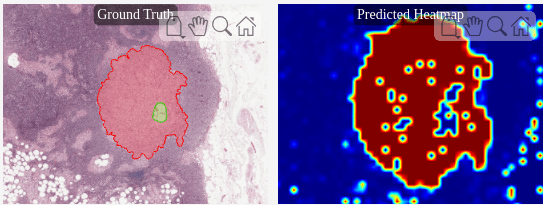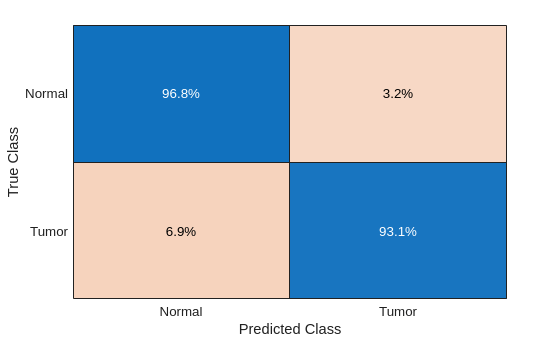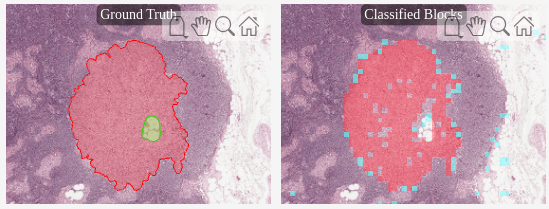# Classify Tumors in Multiresolution Blocked Images

This example shows how to classify multiresolution whole slide images (WSIs) that might not fit in memory using an Inception-v3 deep neural network.

Deep learning methods for tumor classification rely on digital pathology, in which whole tissue slides are imaged and digitized. The resulting WSIs have high resolution, on the order of 200,000-by-100,000 pixels. WSIs are frequently stored in a multiresolution format to facilitate efficient display, navigation, and processing of images.

The example outlines an architecture to use block based processing to train large WSIs. The example trains an Inception-v3 based network using transfer learning techniques to classify individual blocks as `normal` or `tumor`.

If you do not want to download the training data and train the network, then continue to the Train or Download Network section of this example.

### Prepare Training Data

Prepare the training and validation data by following the instructions in Preprocess Multiresolution Images for Training Classification Network. The preprocessing example saves the preprocessed training and validation datastores in the a file called `trainingAndValidationDatastores.mat`.

Set the value of the `dataDir` variable as the location where the `trainingAndValidationDatastores.mat` file is located. Load the training and validation datastores into variables called `dsTrainLabeled` `and` `dsValLabeled`.

```dataDir = fullfile(tempdir,"Camelyon16"); load(fullfile(dataDir,"trainingAndValidationDatastores.mat"))```

### Set Up Inception-v3 Network Layers For Transfer Learning

This example uses an Inception-v3 network , a convolutional neural network that is trained on more than a million images from the ImageNet database . The network is 48 layers deep and can classify images into 1,000 object categories, such as keyboard, mouse, pencil, and many animals.

The `inceptionv3` (Deep Learning Toolbox) function returns a pretrained Inception-v3 network. Inception-v3 requires the Deep Learning Toolbox™ Model for Inception-v3 Network support package. If this support package is not installed, then the function provides a download link.

```net = inceptionv3; lgraph = layerGraph(net);```

The convolutional layers of the network extract image features. The last learnable layer and the final classification layer classify an input image using the image features. These two layers contain information on how to combine the features into class probabilities, a loss value, and predicted labels. To retrain a pretrained network to classify new images, replace these two layers with new layers adapted to the new data set. For more information, see Train Deep Learning Network to Classify New Images (Deep Learning Toolbox).

Find the names of the two layers to replace using the helper function `findLayersToReplace`. This function is attached to the example as a supporting file. In Inception-v3, these two layers are named "`predictions`" and "`ClassificationLayer_predictions`".

`[learnableLayer,classLayer] = findLayersToReplace(lgraph);`

The goal of this example is to perform binary segmentation between two classes, `tumor` and `normal`. Create a new fully connected layer for two classes. Replace the final fully connected layer with the new layer.

```numClasses = 2; newLearnableLayer = fullyConnectedLayer(numClasses,Name="predictions"); lgraph = replaceLayer(lgraph,learnableLayer.Name,newLearnableLayer);```

Create a new classification layer for two classes. Replace the final classification layer with the new layer.

```newClassLayer = classificationLayer(Name="ClassificationLayer_predictions"); lgraph = replaceLayer(lgraph,classLayer.Name,newClassLayer);```

### Specify Training Options

Train the network using root mean squared propagation (RMSProp) optimization. Specify the hyperparameter settings for RMSProp by using the `trainingOptions` (Deep Learning Toolbox) function.

Reduce `MaxEpochs` to a small number because the large amount of training data enables the network to reach convergence sooner. Specify a `MiniBatchSize` according to your available GPU memory. While larger mini-batch sizes can make the training faster, larger sizes can reduce the ability of the network to generalize. Set `ResetInputNormalization` to `false` to prevent a full read of the training data to compute normalization stats.

```options = trainingOptions("rmsprop", ... MaxEpochs=1, ... MiniBatchSize=256, ... Shuffle="every-epoch", ... ValidationFrequency=250, ... InitialLearnRate=1e-4, ... SquaredGradientDecayFactor=0.99, ... ResetInputNormalization=false, ... Plots="training-progress");```

By default, this example downloads a pretrained version of the trained classification network using the helper function `downloadTrainedCamelyonNet`. The pretrained network can be used to run the entire example without waiting for training to complete.

To train the network, set the `doTraining` variable in the following code to `true`. Train the network using the `trainNetwork` (Deep Learning Toolbox) function.

Train on one or more GPUs, if available. Using a GPU requires Parallel Computing Toolbox™ and a CUDA® enabled NVIDIA® GPU. For more information, see GPU Support by Release (Parallel Computing Toolbox).

```doTraining =false; if doTraining checkpointsDir = fullfile(dataDir,"checkpoints"); if ~exist(checkpointsDir,"dir") mkdir(checkpointsDir); end options.CheckpointPath=checkpointsDir; options.ValidationData=dsValLabeled; trainedNet = trainNetwork(dsTrainLabeled,lgraph,options); modelDateTime = string(datetime("now",Format="yyyy-MM-dd-HH-mm-ss")); save(dataDir+"trainedCamelyonNet-"+modelDateTime+".mat","trainedNet"); else trainedCamelyonNet_url = "https://www.mathworks.com/supportfiles/vision/data/trainedCamelyonNet.mat"; dataDir = fullfile(tempdir,"Camelyon16"); downloadTrainedNetwork(trainedCamelyonNet_url,dataDir); load(fullfile(dataDir,"trainedCamelyonNet.mat")); end```

The Camelyon16 test data set consists of 130 WSIs. These images have both normal and tumor tissue. The size of each file is approximately 2 GB.

To download the test data, go to the Camelyon17 website and click the first "CAMELYON16 data set" link. Open the "testing" directory, then follow these steps.

• Download the "lesion_annotations.zip" file. Extract all files to the directory specified by the `testAnnotationDir` variable.

• Open the "images" directory. Download the files to the directory specified by the `testImageDir` variable.

```testDir = fullfile(dataDir,"testing"); testImageDir = fullfile(testDir,"images"); testAnnotationDir = fullfile(testDir,"lesion_annotations"); if ~exist(testDir,"dir") mkdir(testDir); mkdir(fullfile(testDir,"images")); mkdir(fullfile(testDir,"lesion_annotations")); end```

### Preprocess Test Data

#### Create `blockedImage` Objects to Manage Test Images

Get the file names of the test images. Then, create an array of `blockedImage` objects that manage the test images. Each `blockedImage` object points to the corresponding image file on disk.

```testFileSet = matlab.io.datastore.FileSet(testImageDir+filesep+"test*"); testImages = blockedImage(testFileSet);```

Set the spatial referencing for all training data by using the `setSpatialReferencingForCamelyon16` helper function. This function is attached to the example as a supporting file. The `setSpatialReferencingForCamelyon16` function sets the `WorldStart` and `WorldEnd` properties of each `blockedImage` object using the spatial referencing information from the TIF file metadata.

`testImages = setSpatialReferencingForCamelyon16(testImages);`

To process the WSI data efficiently, create a tissue mask for each test image. This process is the same as the one used for the preprocessing the normal training images. For more information, see Preprocess Multiresolution Images for Training Classification Network.

```normalMaskLevel = 8; testDir = fullfile(dataDir,"testing"); testTissueMaskDir = fullfile(testDir,"test_tissue_mask_level"+num2str(normalMaskLevel)); if ~isfolder(testTissueMaskDir) testTissueMasks = apply(testImages, @(bs)im2gray(bs.Data)<150, ... BlockSize=[512 512], ... Level=normalMaskLevel, ... UseParallel=canUseGPU, ... DisplayWaitbar=false, ... OutputLocation=testTissueMaskDir); save(fullfile(testTissueMaskDir,"testTissueMasks.mat"),"testTissueMasks") else % Load previously saved data load(fullfile(testTissueMaskDir,"testTissueMasks.mat"),"testTissueMasks"); end```

The tissue masks have only one level and are small enough to fit in memory. Display the tissue masks in the Image Browser app using the `browseBlockedImages` helper function. This helper function is attached to the example as a supporting file.

`browseBlockedImages(testTissueMasks,1);`#### Preprocess Tumor Ground Truth Images

Specify the resolution level of the tumor masks.

`tumorMaskLevel = 8;`

Create a tumor mask for each ground truth tumor image using the `createMaskForCamelyon16TumorTissue` helper function. This helper function is attached to the example as a supporting file. The function performs these operations for each image:

• Read the (x, y) boundary coordinates for all ROIs in the annotated XML file.

• Separate the boundary coordinates for tumor and normal tissue ROIs into separate cell arrays.

• Convert the cell arrays of boundary coordinates to a binary blocked image using the `polyToBlockedImage` function. In the binary image, the ROI indicates tumor pixels and the background indicates normal tissue pixels. Pixels that are within both tumor and normal tissue ROIs are classified as background.

```testTumorMaskDir = fullfile(testDir,['test_tumor_mask_level' num2str(tumorMaskLevel)]); if ~isfolder(testTumorMaskDir) testTumorMasks = createMaskForCamelyon16TumorTissue(testImages,testAnnotationDir,testTumorMaskDir,tumorMaskLevel); save(fullfile(testTumorMaskDir,"testTumorMasks.mat"),"testTumorMasks") else load(fullfile(testTumorMaskDir,"testTumorMasks.mat"),"testTumorMasks"); end```

### Predict Heatmaps of Tumor Probability

Use the trained classification network to predict a heatmap for each test image. The heatmap gives a probability score that each block is of the `tumor` class. The example performs these operations for each test image to create a heatmap:

• Select blocks using the `selectBlockLocations` function. Include all blocks that have at least one tissue pixel by specifying the `InclusionThreshold` name-value argument as `0`.

• Process batches of blocks using the `apply` function with the processing operations defined by the `predictBlock` helper function. The helper function is attached to the example as a supporting file. The `predictBlock` helper function calls the `predict` (Deep Learning Toolbox) function on a block of data and returns the probability score that the block is `tumor`.

• Write the heatmap data to a TIF file using the `write` function. The final output after processing all blocks is a heatmap showing the probability of finding tumors over the entire WSI.

```numTest = numel(testImages); outputHeatmapsDir = fullfile(testDir,"heatmaps"); networkBlockSize = [299,299,3]; tic for ind = 1:numTest % Check if TIF file already exists [~,id] = fileparts(testImages(ind).Source); outFile = fullfile(outputHeatmapsDir,id+".tif"); if ~exist(outFile,"file") bls = selectBlockLocations(testImages(ind),Levels=1, ... BlockSize=networkBlockSize, ... Mask=testTissueMasks(ind),InclusionThreshold=0); % Resulting heat maps are in-memory blockedImage objects bhm = apply(testImages(ind),@(x)predictBlockForCamelyon16(x,trainedNet), ... Level=1,BlockLocationSet=bls,BatchSize=128, ... PadPartialBlocks=true,DisplayWaitBar=false); % Write results to a TIF file write(bhm,outFile,BlockSize=[512 512]); end end toc```

Collect all of the written heatmaps as an array of `blockedImage` objects.

```heatMapFileSet = matlab.io.datastore.FileSet(outputHeatmapsDir,FileExtensions=".tif"); bheatMapImages = blockedImage(heatMapFileSet);```

#### Visualize Heatmap

Select a test image to display. On the left side of a figure, display the ground truth boundary coordinates as freehand ROIs using the `showCamelyon16TumorAnnotations` helper function. This helper function is attached to the example as a supporting file. Normal regions (shown with a green boundary) can occur inside tumor regions (shown with a red boundary).

```idx = 27; figure tiledlayout(1,2) nexttile hBim1 = showCamelyon16TumorAnnotations(testImages(idx),testAnnotationDir); title("Ground Truth")```

On the right side of the figure, display the heatmap for the test image.

```nexttile hBim2 = bigimageshow(bheatMapImages(idx),Interpolation="nearest"); colormap(jet)```

Link the axes and zoom in to an area of interest.

```linkaxes([hBim1.Parent,hBim2.Parent]) xlim([53982, 65269]) ylim([122475, 133762]) title("Predicted Heatmap")```### Classify Test Images at Specific Threshold

To classify blocks as `tumor` or `normal`, apply a threshold to the heatmap probability values.

Pick a threshold probability above which blocks are classified as `tumor`. Ideally, you would calculate this threshold value using receiver operating characteristic (ROC) or precision-recall curves on the validation data set.

`thresh = 0.8;`

Classify the blocks in each test image and calculate the confusion matrix using the `apply` function with the processing operations defined by the `computeBlockConfusionMatrixForCamelyon16` helper function. The helper function is attached to the example as a supporting file.

The `computeBlockConfusionMatrixForCamelyon16` helper function performs these operations on each heatmap:

• Resize and refine the ground truth mask to match the size of the heatmap.

• Apply the threshold on the heatmap.

• Calculate a confusion matrix for all of the blocks at the finest resolution level. The confusion matrix gives the number of true positive (TP), false positive (FP), true negative (TN), and false negative (FN) classification predictions.

• Save the total counts of TP, FP, TN, and FN blocks as a structure in a blocked image. The blocked image is returned as an element in the array of blocked images, `bcmatrix`.

• Save a numeric labeled image of the classification predictions in a blocked image. The values 0, 1, 2, and 3 correspond to TN, FP, FN, and TP results, respectively. The blocked image is returned as an element in the array of blocked images, `bcmatrixImage`.

```for ind = 1:numTest [bcmatrix(ind),bcmatrixImage{ind}] = apply(bheatMapImages(ind), ... @(bs,tumorMask,tissueMask)computeBlockConfusionMatrixForCamelyon16(bs,tumorMask,tissueMask,thresh), ... ExtraImages=[testTumorMasks(ind),testTissueMasks(ind)]); end```

Calculate the global confusion matrix over all test images.

```cmArray = arrayfun(@(c)gather(c),bcmatrix); cm = [sum([cmArray.tp]),sum([cmArray.fp]); sum([cmArray.fn]),sum([cmArray.tn])];```

Display the confusion chart of the normalized global confusion matrix. The majority of blocks in the WSI images are of normal tissue, resulting in a high percentage of true negative predictions.

```figure confusionchart(cm,["Tumor","Normal"],Normalization="total-normalized")```#### Visualize Classification Results

Compare the ground truth ROI boundary coordinates with the classification results. On the left side of a figure, display the ground truth boundary coordinates as freehand ROIs. On the right side of the figure, display the test image and overlay a color on each block based on the confusion matrix. Display true positives as red, false positives as cyan, false negatives as yellow, and true negatives with no color.

False negatives and false positives appear around the edges of the tumor region, which indicates that the network has difficulty classifying blocks with partial classes.

```idx = 27; figure tiledlayout(1,2) nexttile hBim1 = showCamelyon16TumorAnnotations(testImages(idx),testAnnotationDir); title("Ground Truth") nexttile hBim2 = bigimageshow(testImages(idx)); cmColormap = [0 0 0; 0 1 1; 1 1 0; 1 0 0]; showlabels(hBim2,bcmatrixImage{idx}, ... Colormap=cmColormap,AlphaData=bcmatrixImage{idx}) title("Classified Blocks") linkaxes([hBim1.Parent,hBim2.Parent]) xlim([56000 63000]) ylim([125000 132600])```Note: To reduce the classification error around the perimeter of the tumor, you can retrain the network with less homogenous blocks. When preprocessing the `Tumor` blocks of the training data set, reduce the value of the `InclusionThreshold` name-value argument.

### Quantify Network Prediction with AUC-ROC Curve

Calculate the ROC curve values at different thresholds by using the `computeROCCurvesForCamelyon16` helper function. This helper function is attached to the example as a supporting file.

```threshs = [1 0.99 0.9:-.1:.1 0.05 0]; [tpr,fpr,ppv] = computeROCCurvesForCamelyon16(bheatMapImages,testTumorMasks,testTissueMasks,threshs);```

Calculate the area under the curve (AUC) metric using the `trapz` function. The metric returns a value in the range [0, 1], where 1 indicates perfect model performance. The AUC for this data set is close to 1. You can use the AUC to fine-tune the training process.

```figure stairs(fpr,tpr,"-"); ROCAUC = trapz(fpr,tpr); title(["Area Under Curve: " num2str(ROCAUC)]); xlabel("False Positive Rate") ylabel("True Positive Rate")```### References

 Ehteshami Bejnordi, Babak, Mitko Veta, Paul Johannes van Diest, Bram van Ginneken, Nico Karssemeijer, Geert Litjens, Jeroen A. W. M. van der Laak, et al. “Diagnostic Assessment of Deep Learning Algorithms for Detection of Lymph Node Metastases in Women With Breast Cancer.” JAMA 318, no. 22 (December 12, 2017): 2199–2210. https://doi.org/10.1001/jama.2017.14585.

 Szegedy, Christian, Vincent Vanhoucke, Sergey Ioffe, Jonathon Shlens, and Zbigniew Wojna. “Rethinking the Inception Architecture for Computer Vision.” Preprint, submitted December 2, 2015. https://arxiv.org/abs/1512.00567v3.

 ImageNet. https://www.image-net.org.